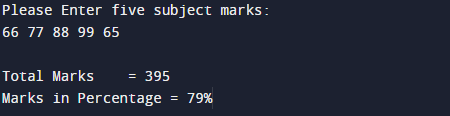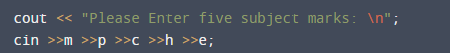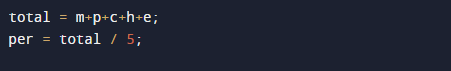# C++ Program to Calculate Percentage Of Students Marks

In this tutorial you will learn about the C++ Program to Calculate Percentage Of Students Marks and its application with practical example.

In this tutorial, we will learn to create a c++ program that will calculate Percentage Of students using c++ programming

## Prerequisites

Before starting with this program  we assume that you are best aware of the following C ++ programming topics:

• Operators.
• Basic input/output.
• Basic c++ programming.

## C++ Program to Calculate entered 5 subjects marks and calculate percentage.

In this program we will create a program in which user enter the marks of five subjects and calculate percentage.We would first declared and initialized the required variables. Next, we would prompt user to input the number of terms. Later we will calculate percentage of given number of terms.
And you will learn how to find percentage of the five subjects in C++ language.
using for loop.

## OutputIn the above program, we have first declared and initialized a set variables required in the program.

• m = holds value of math.
• p = holds value of physics.
• c = holds value of chemistry
• h = holds value of Hindi.
• e= holds value of English.
• total = calculate sum of value.
• per= it will hold percentage.

In this  C++ program to calculate Percentage of Five Subjects .

In the first  cout<<“” statement will ask the user to enter the 5 subjects number.then we add all 5 given  value  in variable total ( total =m+p+c+h+e ).Now, we calculated the percentage (per=total/5).

And we will get the required output.This program allows user to enter five values of five different  subjects. And then calculate the Total and Percentage of those Five Subjects.

In this tutorial we have learn about the C++ Program to Calculate Percentage Of Students Marks and its application with practical example. I hope you will like this tutorial.